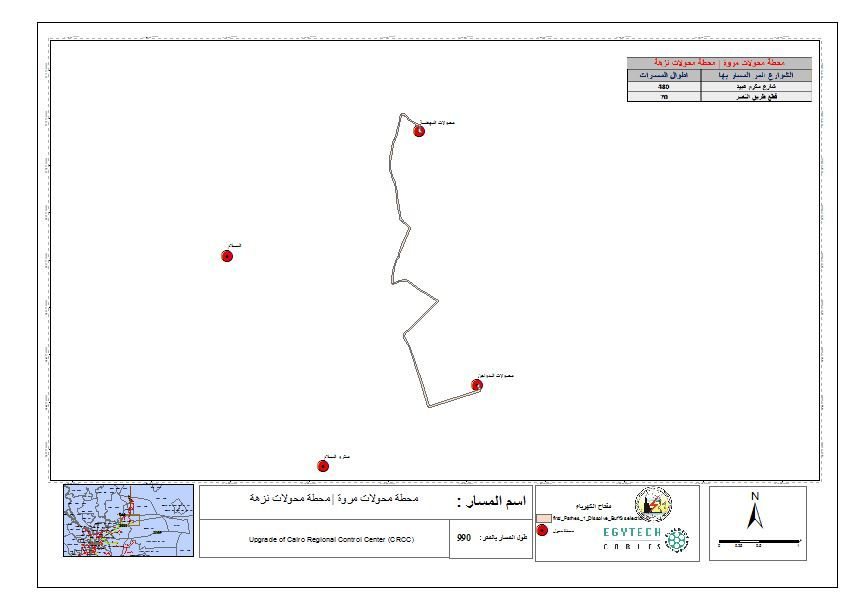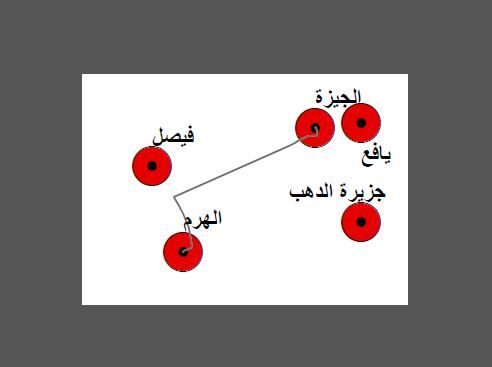# export map layout with loop definition query

1241
22
09-15-2021 02:08 AMNew Contributor III

i would like to complete my script to zoom to layer per definition and export pdf but the script give me bugs when it export pdf and not zooming can any help me

import arcpy, os

arcpy.env.overwriteOutput = True
mxd = arcpy.mapping.MapDocument("CURRENT")
df = arcpy.mapping.ListDataFrames(mxd)

layerName = arcpy.GetParameterAsText(0)
fieldName = arcpy.GetParameterAsText(1)
imgLocation = arcpy.GetParameterAsText(2)
whereList = []

layerFile = arcpy.mapping.Layer(layerName)
layerFile.definitionQuery = ""

print df.name

with arcpy.da.SearchCursor(layerName, fieldName) as cursor:
for row in cursor:
for item in row:
whereList.append(item)

for wl in whereList:
layerFile.definitionQuery = fieldName + "= '" + wl + "'"
df = layerFile.getExtent()
df.extent = outFile
outFile = imgLocation + "\\" + wl + ".pdf"

arcpy.mapping.ExportToPDF(mxd, outFile)

del mxd

Tags (4)
7 Solutions

Accepted SolutionsbyMVP Frequent Contributor

This bit makes no sense

``````#variables are all messed up
for wl in whereList:
layerFile.definitionQuery = fieldName + "= '" + wl + "'"
df = layerFile.getExtent()
df.extent = outFile
outFile = imgLocation + "\\" + wl + ".pdf"

#should be
for wl in whereList:
layerFile.definitionQuery = fieldName + "= '" + wl + "'"
new_extent = layerFile.getExtent()
df.extent = new_extent
arcpy.RefreshActiveView()
outFile = imgLocation + "\\" + wl + ".pdf"``````New Contributor III

the extent run very well but still getting error to export pdf

File "c:\program files (x86)\arcgis\desktop10.4\arcpy\arcpy\mapping.py", line 1156, in ExportToPDF
layout.exportToPDF(*args)
AttributeError: Invalid destination path

Failed to execute (Script)byMVP Frequent Contributor

what path are you supplying for imgLocation?

I prefer to use the os.path.join() method when constructing paths:

``````outFile = os.path.join(imgLocation, wl + ".pdf")byMVP Frequent Contributor

probably adjust the scale factor e.g.

``df.scale *= 1.15``byMVP Frequent Contributor

Can you share your final code?

You're saying that the second image is the pdf produced?

Are you sure you're specifying the same MXD which contains the layout?

The layout should be used as default in arcpy.mapping.ExportToPDF(mxd, outFile), but maybe try this just in case:

``arcpy.mapping.ExportToPDF(mxd, outFile, "PAGE_LAYOUT")``

and what if you try JPEG or PNG etc. ?

``````outFile = os.path.join(imgLocation, wl + ".jpg")
arcpy.mapping.ExportToJPEG(mxd, outFile)``````byMVP Frequent Contributor

ah I think I made a silly error, I'm unable to test this.

side note - why are you using mxd.save() and why have you got outFile variable created then overwritten 2 lines later?

``````import arcpy, os

arcpy.env.overwriteOutput = True
mxd = arcpy.mapping.MapDocument("CURRENT")

df = arcpy.mapping.ListDataFrames(mxd)

layerName = arcpy.GetParameterAsText(0)
fieldName = arcpy.GetParameterAsText(1)
imgLocation = arcpy.GetParameterAsText(2)
whereList = []

layerFile = arcpy.mapping.Layer(layerName)
layerFile.definitionQuery = ""

print df.name

with arcpy.da.SearchCursor(layerName, fieldName) as cursor:
for row in cursor:
for item in row:
whereList.append(item)

for wl in whereList:

arcpy.RefreshActiveView()
mxd.save()
layerFile.definitionQuery = fieldName + "= '" + wl + "'"
new_extent = layerFile.getExtent()
df.extent = new_extent
df.scale *= 1.5

for elm in arcpy.mapping.ListLayoutElements(mxd, "TEXT_ELEMENT", "title"):

if elm.name == "title":
elm.text = wl

arcpy.RefreshActiveView()
outFile = os.path.join(imgLocation, wl + ".pdf")
outFile = imgLocation + "\\" + wl + ".pdf"
arcpy.mapping.ExportToPDF(mxd, outFile, "PAGE_LAYOUT")
del mxd``````byMVP Frequent Contributor

.strip() would only remove trailing and leading stuff.  Probably best to do something like this:

``````name = ''
for char in wl:
if char.isalpha():
name+=char
outFile = imgLocation + '\\' + name + '.pdf'``````
22 RepliesbyMVP Frequent Contributor

This bit makes no sense

``````#variables are all messed up
for wl in whereList:
layerFile.definitionQuery = fieldName + "= '" + wl + "'"
df = layerFile.getExtent()
df.extent = outFile
outFile = imgLocation + "\\" + wl + ".pdf"

#should be
for wl in whereList:
layerFile.definitionQuery = fieldName + "= '" + wl + "'"
new_extent = layerFile.getExtent()
df.extent = new_extent
arcpy.RefreshActiveView()
outFile = imgLocation + "\\" + wl + ".pdf"``````New Contributor III

the extent run very well but still getting error to export pdf

File "c:\program files (x86)\arcgis\desktop10.4\arcpy\arcpy\mapping.py", line 1156, in ExportToPDF
layout.exportToPDF(*args)
AttributeError: Invalid destination path

Failed to execute (Script)byMVP Frequent Contributor

what path are you supplying for imgLocation?

I prefer to use the os.path.join() method when constructing paths:

``````outFile = os.path.join(imgLocation, wl + ".pdf")New Contributor III

it worked thanks but when it get extent , the extent 100% i want to customize it to 115% to  make the feature fully visiblebyMVP Frequent Contributor

probably adjust the scale factor e.g.

``df.scale *= 1.15``New Contributor III

how i export the pdf from the layout ?New Contributor IIIthis my layout and after i export the layout to pdf after i export it looked thisand the size page is A1byMVP Frequent Contributor

Can you share your final code?

You're saying that the second image is the pdf produced?

Are you sure you're specifying the same MXD which contains the layout?

The layout should be used as default in arcpy.mapping.ExportToPDF(mxd, outFile), but maybe try this just in case:

``arcpy.mapping.ExportToPDF(mxd, outFile, "PAGE_LAYOUT")``

and what if you try JPEG or PNG etc. ?

``````outFile = os.path.join(imgLocation, wl + ".jpg")
arcpy.mapping.ExportToJPEG(mxd, outFile)``````New Contributor III

you helped me more thanks this the final script by DavidPike
i want use the dynamic table and this not in armap this onlyy in arcgis pro so i want convert my final script to arcgis pro

import arcpy, os

arcpy.env.overwriteOutput = True
mxd = arcpy.mapping.MapDocument("CURRENT")

df = arcpy.mapping.ListDataFrames(mxd)

layerName = arcpy.GetParameterAsText(0)
fieldName = arcpy.GetParameterAsText(1)
imgLocation = arcpy.GetParameterAsText(2)
whereList = []

layerFile = arcpy.mapping.Layer(layerName)
layerFile.definitionQuery = ""

print df.name

with arcpy.da.SearchCursor(layerName, fieldName) as cursor:
for row in cursor:
for item in row:
whereList.append(item)

for wl in whereList:
layerFile.definitionQuery = fieldName + "= '" + wl + "'"

new_extent = layerFile.getExtent()
df.extent = new_extent
df.scale *= 1.5
arcpy.RefreshActiveView()
outFile = os.path.join(imgLocation, wl + ".pdf")